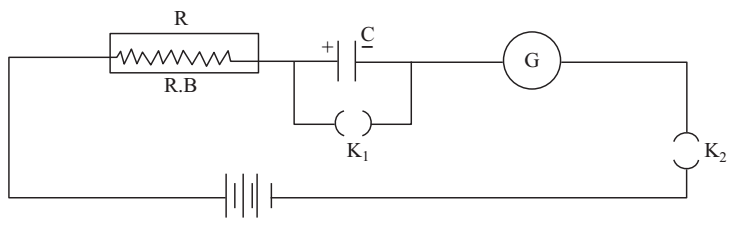# Time Constant of RC Circuit Experiment

Experiment: Study decay of current in a RC circuit while charging the capacitor, using a galvanometer and find the time constant of the circuit.

Capacitance C of a capacitor is defined as the ratio of charge Q to electric potential difference V.

Each capacitor is characterized by its capacitance and the maximum voltage it can withstand. Thus, a 1000 μF 10 V capacitor can be charged to a maximum potential difference of 10 volts.

Let the capacitor be uncharged initially. On closing the key at i = 0 the charging current I in the circuit decreases exponentially with time from its initial value Io = V/R to zero as

I = Ioe-t/RC

In a time t = RC, called the time constant, the charging current becomes I = Io/e = Io/2.72

I - 0.37Io

Also, I = Io/2 in a time t = T1/2 (called half-life).

Material Required

Electrolytic capacitor 2000 μF 10V, a high value resistance box RB(H). A 3V/9V battery, single keys, a stop watch, connecting wires, sand paper.

### How To Set Up

Clean the ends of the connecting wires with the help of sand paper. Connect the circuit.While making connections take care to connect the positive terminal of the 2000 μF 10V capacitor to the positive terminal of the battery. Also take care to remove plugs from the keys before connecting them in the circuit.

### How To Perform Experiment

1. Check the circuit for the connection by comparing it with the circuit diagram.

2. Note that the galvanometer pointer is at ‘0’. If needed, adjust it to bring it to 0 (consult Laboratory technician).

3. Close key K1.

4. Introduce a high value resistance (say 10000 Ω) in the circuit from the resistance box. This is done to keep the galvanometer deflection within its scale when key K2 is closed.

5. Now close key K2 also, and adjust the resistance box to get full scale deflection in the galvanometer. Record R, the resistance offered by the resistance box in the observation table.

6. Keep the stop watch ready. As you remove the plug from the key K1 start the stop watch at the same time (say t = 0). From this time onward the capacitor will start charging.

7. Record the time every time when the galvanometer deflection becomes 24 divisions, 20 divisions,  16 divisions, ..., 4 divisions, 3 divisions, 2 divisions.

8. Plot galvanometer deflection θ along y-axis against time t along x axis.

9. From the graph find T1/2 the “time” in which the galvanometer deflection reduces to 50% of its initial value and compare it with 0.69RC. Choose several initial values e.g. 30 div., 20 div., 16 div. 10 div., 6 div. For each initial value find T1/2. If these value of T1/2 are equal within experimental error, find their mean.

10. From the graph find the time in which the charging current hence galvanometer deflection) falls to 0.368 times its initial value i.e. θ falls from initial value of 30 div to (.362) (30) i.e. 11 divisions, or 22 divisions to 8 divisions, or 16 to 6, or 11 to 4, approximately and compare it with RC.

11. The value of RC i.e. time constant should be sufficiently large to record the observations properly.

### Sources of Error

1. There may be some error in the calculation of RC time constant and T1/2 = 0.693 RC on account of the following:
• R should also include galvanometer resistance which though small may not be negligible.
• Electrolytic capacitors have large tolerance i.e. their actual capacitance may differ from the printed value by 20%.
2. There may be error in recording galvanometer deflection and in recording time using stop watch on account of finite least count of these instruments.